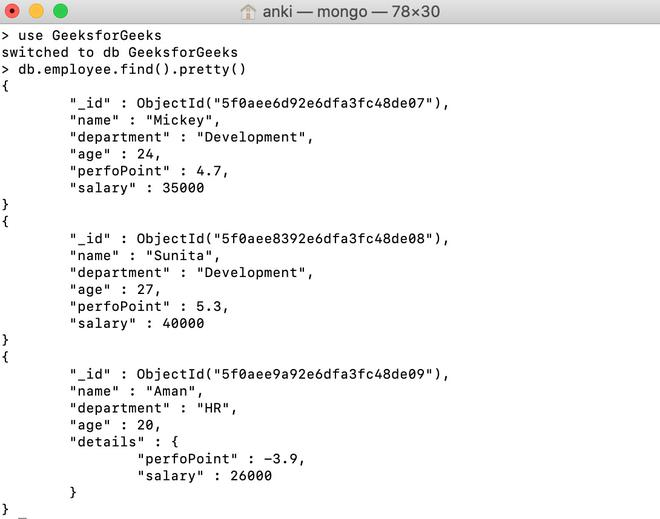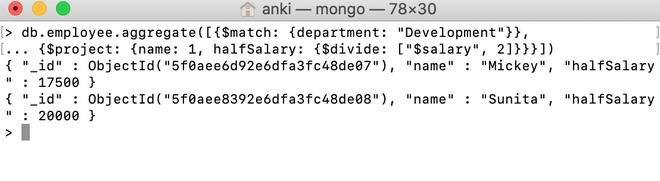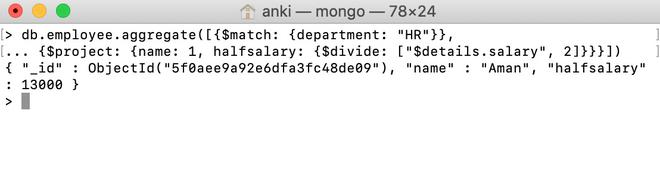# MongoDB \$divide Operator

• Last Updated : 28 Jul, 2020

MongoDB provides different types of arithmetic expression operators that are used in the aggregation pipeline stages and \$divide operator is one of them. This operator is used to divide one number by another number and return the result of the division.

Syntax:

```{ \$divide: [ <expression1>, <expression2> ] }
```

Here, in this operator, the arguments passed in an array.  And the first argument is a dividend and the second argument is a divisor.  The argument must be a valid expression until it resolves to a number.

Examples:

In the following examples, we are working with:

Database: GeeksforGeeks

Collection: employee

Document: three documents that contain the details of the employees in the form of field-value pairs.Divide using \$divide operator:

In this example, we are going to divide the salary of the development department’s employees into half.

```db.employee.aggregate([{\$match: {department: "Development"}},
... {\$project: {name: 1, halfSalary: {\$divide: ["\$salary", 2]}}}])

```Divide using \$divide operator in the embedded document:

In this example, we are going to divide the salary of the HR department’s employees into half.

```db.employee.aggregate([{\$match: {department: "HR"}},
... {\$project: {name: 1, halfsalary:
{\$divide: ["\$details.salary", 2]}}}])

```My Personal Notes arrow_drop_up